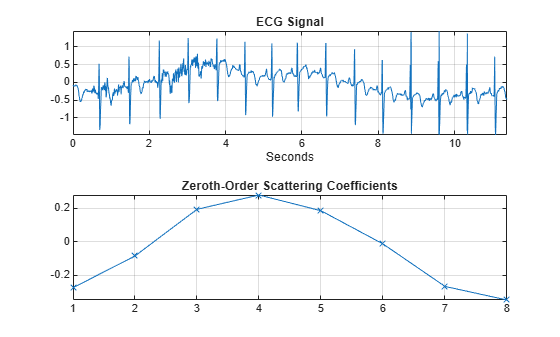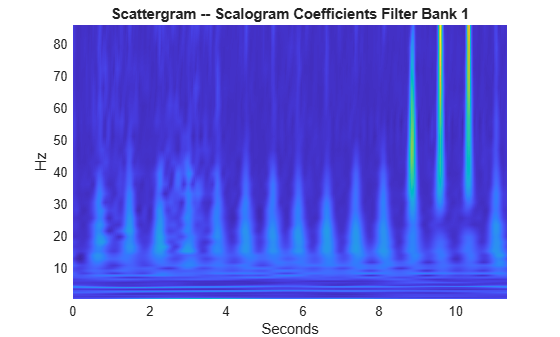# scatteringTransform

Wavelet 1-D scattering transform

## Syntax

``s = scatteringTransform(sf,x)``
``[s,u] = scatteringTransform(sf,x)``

## Description

````s = scatteringTransform(sf,x)` returns the wavelet 1-D scattering transform of `x` with metadata for the wavelet time scattering network, `sf`. `x` is a real-valued vector, matrix, or 3-D array.The precision of the scattering coefficients depends on the precision specified in the scattering network `sf`.```

example

````[s,u] = scatteringTransform(sf,x)` also returns the scalogram coefficients for each of the scattering orders.The precision of the scalogram coefficients depends on the precision specified in the scattering network `sf`.```

## Examples

collapse all

This example shows how to return the wavelet 1-D scattering transform of a real-valued signal.

Load an ECG signal sampled at 180 Hz.

```load wecg Fs = 180;```

Create a wavelet time scattering network to apply to the signal. Compute the scattering transform of the signal.

```sf = waveletScattering('SignalLength',numel(wecg),... 'SamplingFrequency',Fs)```
```sf = waveletScattering with properties: SignalLength: 2048 InvarianceScale: 5.6889 QualityFactors: [8 1] Boundary: 'periodic' SamplingFrequency: 180 Precision: 'double' OversamplingFactor: 0 OptimizePath: 0 ```
`[S,U] = scatteringTransform(sf,wecg);`

Plot the signal and the zeroth-order scattering coefficients. Note that the invariance scale is one half the duration of the signal.

```t = [0:length(wecg)-1]/Fs; subplot(2,1,1) plot(t,wecg) grid on axis tight xlabel('Seconds') title('ECG Signal') subplot(2,1,2) plot(S{1}.signals{1},'x-') grid on axis tight title('Zeroth-Order Scattering Coefficients')```Visualize the scattergram for the first-order scalogram coefficients.

```figure scattergram(sf,U,'FilterBank',1)```## Input Arguments

collapse all

Wavelet time scattering network, specified as a `waveletScattering` object.

Input data, specified as a real-valued vector, matrix, or 3-D array. If `x` is a vector, the number of samples in `x` must equal the `SignalLength` value of `sf`. If `x` is a matrix or 3-D array, the number of rows in `x` must equal the `SignalLength` value of `sf`. If `x` is 2-D, the first dimension is assumed to be time and the columns of `x` are assumed to be separate channels. If `x` is 3-D, the dimensions of `x` are Time-by-Channel-by-Batch.

Data Types: `single` | `double`

## Output Arguments

collapse all

Scattering coefficients, returned as a NO-by-1 cell array, where NO is the number of orders in `sf`.

Each element of `s` is a MATLAB® table with the following variables:

Scattering coefficients, returned as a cell array. If `x` is a vector, each element of `signals` is a Ns-by-1 vector, where Ns is the number of scattering coefficients. If `x` is 2-D, each element of `signals` is a Ns-by-Nc matrix, where Nc is the number of channels in `x`. If `x` is 3-D, each element of `signals` is a Ns-by-Nc-by-Nb array, where Nb is the number of batches in `x`.

Data Types: `single` | `double`

Scattering path used to obtain the scattering coefficients, returned as a row vector. Each column of `path` corresponds to one element of the path. The scalar 0 denotes the original signal. Positive integers in the Lth column denote the corresponding wavelet filter in the (L-1)th filter bank. Wavelet bandpass filters are ordered by decreasing center frequency.

Data Types: `double`

Bandwidth of the scattering coefficients, returned as a scalar. If you specify a sampling frequency in the scattering network, the bandwidth is in hertz. Otherwise, the bandwidth is in cycles/sample.

Data Types: `double`

Base-2 log resolution of the scattering coefficients, returned as a scalar.

Data Types: `double`

Scalogram coefficients, returned as a NO-by-1 cell array, where NO is the number of orders in `sf`. The ith element of `u` are the scalogram coefficients for the ith row of `s`.

Each element of `u` is a MATLAB table with the following variables:

Scalogram coefficients, returned as a cell array. If `x` is a vector, each element of `coefficients` is a Nu-by-1 vector, where Nu is the number of scalogram coefficients. If `x` is 2-D, each element of `coefficients` is a Nu-by-Nc matrix, where Nc is the number of channels in `x`. If `x` is 3-D, each element of `coefficients` is a Nu-by-Nc-by-Nb array, where Nb is the number of batches in `x`.

Note that `u{1}` contains the original data in the coefficients variable.

Data Types: `single` | `double`

Scattering path used to obtain the scalogram coefficients, returned as a row vector. Each column of `path` corresponds to one element of the path. The scalar 0 denotes the original signal. Positive integers in the Lth column denote the corresponding wavelet filter in the (L-1)th filter bank. Wavelet bandpass filters are ordered by decreasing center frequency.

Data Types: `double`

Bandwidth of the scalogram coefficients, returned as a scalar. If you specify a sampling frequency in the scattering network, the bandwidth is in hertz. Otherwise, the bandwidth is in cycles/sample.

Data Types: `double`

Base-2 log resolution of the scalogram coefficients, returned as a scalar.

Data Types: `double`

## Tips

• The `scatteringTransform` function calls `featureMatrix` to generate the scattering and scalogram coefficients. If you only require the coefficients themselves, for improved performance the recommended approach is to use `featureMatrix`. Use `scatteringTransform` if you are also interested in the coefficients metadata.

## Version History

Introduced in R2018b